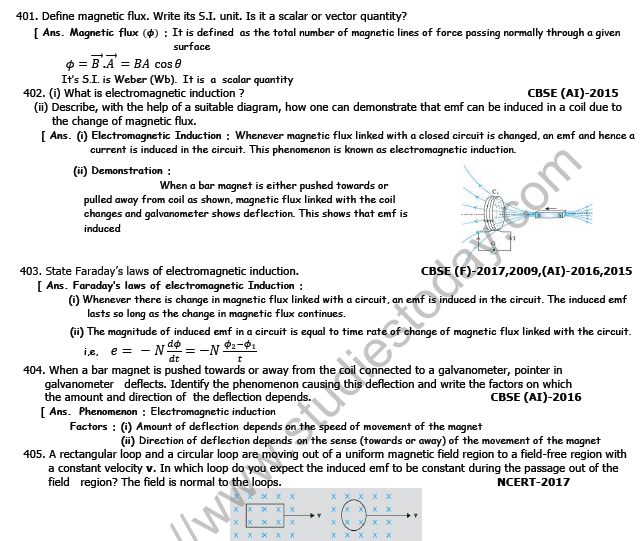# CBSE Class 12 Physics Electromagnetic Induction And Alternating Current Worksheet Set E

Read and download free pdf of CBSE Class 12 Physics Electromagnetic Induction And Alternating Current Worksheet Set E. Students and teachers of Class 12 Physics can get free printable Worksheets for Class 12 Physics in PDF format prepared as per the latest syllabus and examination pattern in your schools. Standard 12 students should practice questions and answers given here for Physics in Grade 12 which will help them to improve your knowledge of all important chapters and its topics. Students should also download free pdf of Class 12 Physics Worksheets prepared by school teachers as per the latest NCERT, CBSE, KVS books and syllabus issued this academic year and solve important problems with solutions on daily basis to get more score in school exams and tests

## Worksheet for Class 12 Physics Chapter 6 Electromagnetic Induction

Class 12 Physics students should refer to the following printable worksheet in Pdf for Chapter 6 Electromagnetic Induction in standard 12. This test paper with questions and answers for Grade 12 Physics will be very useful for exams and help you to score good marks

### Class 12 Physics Worksheet for Chapter 6 Electromagnetic InductionImportant Questions for NCERT Class 12 Physics Electromagnetic Induction

Question. A rectangular coil of 20 turns and area of cross-section 25 sq. cm has a resistance of 100 W. If a magnetic field which is perpendicular to the plane of coil changes at a rate of 1000 tesla per second, the current in the coil is
(a) 1 A
(b) 50 A
(c) 0.5 A
(d) 5 A

Question. A conducting square frame of side ‘a’ and a long straight wire carrying current I are located in the same plane as shown in the figure. The frame moves to the right with a constant velocity ‘V’. The emf induced in the frame will be proportional to
(a) 1/(2x + a)2
(b) 1/(2x − a)(2x + a)
(c) 1/x2
(d) 1/(2x − a)2

Question. If N is the number of turns in a coil, the value of self inductance varies as
(a) N 0
(b) N
(c) N 2
(d) N–2

Question. A magnetic field of 2 × 10–2 T acts at right angles to a coil of area 100 cm2, with 50 turns. The average e.m.f. induced in the coil is 0.1 V, when it is removed from the field in t sec. The value of t is
(a) 10 s
(b) 0.1 s
(c) 0.01 s
(d) 1 s

Question. An electron moves on a straight line path XY as shown. The abcd is a coil adjacent to the path of electron. What will be the direction of current, if any, induced in the coil?
(a) The current will reverse its direction as the electron goes past the coil
(b) No current induced
(c) abcd

Question. Two coils of self inductance 2 mH and 8 mH are placed so close together that the effective flux in one coil is completely linked with the other.
The mutual inductance between these coils is
(a) 16 mH
(b) 10 mH
(c) 6 mH
(d) 4 mH

Question. A metal ring is held horizontally and bar magnet is dropped through the ring with its length along the axis of the ring. The acceleration of the falling magnet is
(a) more than g
(b) equal to g
(c) less than g
(d) either(a) or (c)

Question. Faraday’s laws are consequence of conservation of
(a) energy
(b) energy and magnetic field
(c) charge
(d) magnetic field

Question. A conducting rod AB moves parallel to X-axis in a uniform magnetic field, pointing in the positive X-direction. The end A of the rod gets
(a) positively charged
(b) negatively charged
(c) neutral
(d) first positively charged and then negatively charged

Important Questions for NCERT Class 12 Physics Alternating Induction

Question. In an a.c. circuit the e.m.f. (e) and the current (i) at any instant are given respectively by e = E0sinwt , i = I0sin(wt – f)
The average power in the circuit over one cycle of a.c. is
(a) E0I0 /2 cosΦ
(b) E0I0
(c) E0I0/2
(d) E0I0 /2 sinΦ

Question. A coil of inductive reactance 31 W has a resistance of 8 W. It is placed in series with a condenser of capacitative reactance 25 W. The combination is connected to an a.c. source of 110 V. The power factor of the circuit is
(a) 0.33
(b) 0.56
(c) 0.64
(d) 0.80

Question. The mutual inductance of a pair of coils, each of N turns, is M henry. If a current of I ampere in one of the coils is brought to zero in t second, the emf induced per turn in the other coil, in volt, will be A
(a) MI /t
(b) NMI/t
(c) MN /It
(d) MI/Nt

Question. For a series LCR circuit, the power loss at resonance is
(a)  V2 /[ωL - 1/ωC]
(b) I2
(c) I2R
(d)  V2/ Cω

Question. In an a.c. circuit with phase voltage V and current I, the power dissipated is
(a) V.I
(b) depends on phase angle between V and I
(c) 1/2 ×V.I
(d) 1/√2 ×V.I

Question. A step down transformer is connected to 2400 volts line and 80 amperes of current is found to flow in output load. The ratio of the turns in primary and secondary coil is 20 : 1. If transformer efficiency is 100%, then the current flowing in the primary coil will be
(a) 1600 amp
(b) 20 amp
(c) 4 amp
(d) 1.5 amp

Question. An series L-C-R circuit is connected to a source of A.C. current. At resonance, the phase difference between the applied voltage and the current in the circuit, is
(a) p
(b) zero
(c) p/4
(d) p/2